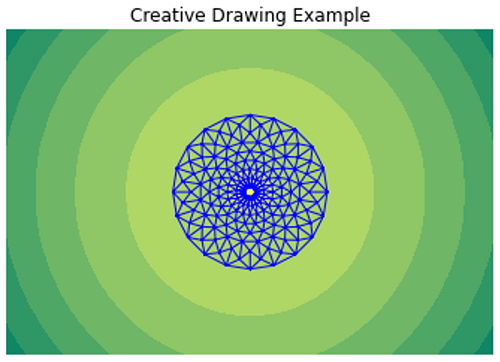# Python | Creative Drawing Example in Matplotlib

In this article, we are presenting a creative drawing example using matplotlib in Python.
Submitted by Anuj Singh, on August 20, 2020

Through this article, we can show that there are different types of images or designs we can create in matplotlib. In the following example, there is a tri-point circular design over a circular uniform contour plot. We have used a summer colormap and blue overlay design color.

The following code shows the implementation of the following design. Moreover, we can change the color combination by using different colormaps such as inferno.

Illustrations:## Python code for creative drawing example in matplotlib

```import matplotlib.pyplot as plt
import numpy as np
from numpy import ma
from matplotlib import ticker, cm
import matplotlib.tri as tri

n_angles = 20

angles = np.linspace(0, 2 * np.pi, n_angles, endpoint=False)
angles = np.repeat(angles[..., np.newaxis], n_radii, axis=1)
angles[:, 1::2] += np.pi / n_angles

triang = tri.Triangulation(x, y)

y[triang.triangles].mean(axis=1))

N = 50
x = np.linspace(-3.0, 3.0, N)
y = np.linspace(-2.0, 2.0, N)

X, Y = np.meshgrid(x, y)

Z1 = np.exp(-X**2 - Y**2)
Z2 = np.exp(-(X * 10)**2 - (Y * 10)**2)
z = Z1 + 50 * Z2
z = ma.masked_where(z <= 0, z)
fig, ax = plt.subplots()
cs = ax.contourf(X, Y, z, locator=ticker.LogLocator(), cmap='summer')
ax.set_aspect('equal')
ax.triplot(triang, 'b-', lw=1)
ax.set_title('Creative Drawing Example')
ax.axis(False)

plt.show()
```

Output:

```Output is as Figure
```